# Hopf fibration

(diff) ← Older revision | Latest revision (diff) | Newer revision → (diff)

A locally trivial fibration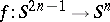for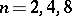. This is one of the earliest examples of locally trivial fibrations, introduced by H. Hopf in . These mappings induce trivial mappings in homology and cohomology; however, they are not homotopic to the null mapping, which follows from the fact that their Hopf invariant is non-trivial. The creation of the mappings requires the so-called Hopf construction.

Let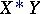be the join of two spacesand, which has natural coordinates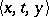, where,,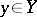. Here, for example,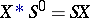, where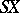is the suspension of. The Hopf construction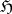associates with a mapping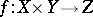the mappinggiven by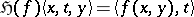.

Suppose that mappings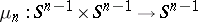are defined forby means of multiplications: in the complex numbers for, in the quaternions for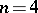, and in the Cayley numbers for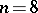. Then, and the Hopf mapping is defined asThe Hopf mapping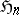,, is a locally trivial fibration with fibre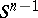. Ifis a mapping of bidegree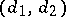, then the Hopf invariant of the mapping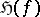is. In particular, the Hopf invariant of the Hopf fibration is 1.

Sometimes the Hopf fibration is defined as the mappinggiven by the formula,. This mapping is a locally trivial fibration with fibre. Forone obtains the classical Hopf fibration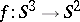.

How to Cite This Entry:
Hopf fibration. Encyclopedia of Mathematics. URL: http://encyclopediaofmath.org/index.php?title=Hopf_fibration&oldid=47269
This article was adapted from an original article by A.V. Shokurov (originator), which appeared in Encyclopedia of Mathematics - ISBN 1402006098. See original article